# PCA （主成分分析）详解 （写给初学者） 结合matlab

2014/04/22 20:58

## （转载请注明出处）

1、原始数据：

x=[2.5, 0.5, 2.2, 1.9, 3.1, 2.3, 2, 1, 1.5, 1.1]T
y=[2.4, 0.7, 2.9, 2.2, 3.0, 2.7, 1.6, 1.1, 1.6, 0.9]T

2、计算协方差矩阵

（1）协方差矩阵：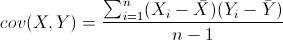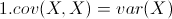(X的方差)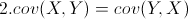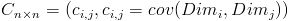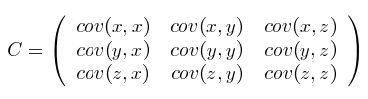（2）协方差矩阵的求法：

MySample = fix(rand(10,3)*50)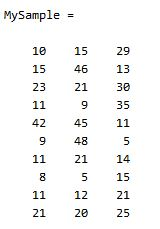dim1 = MySample(:,1);
dim2 = MySample(:,2);
dim3 = MySample(:,3);

sum( (dim1-mean(dim1)) .* (dim2-mean(dim2)) ) / ( size(MySample,1)-1 ) % 得到  74.5333
sum( (dim1-mean(dim1)) .* (dim3-mean(dim3)) ) / ( size(MySample,1)-1 ) % 得到  -10.0889
sum( (dim2-mean(dim2)) .* (dim3-mean(dim3)) ) / ( size(MySample,1)-1 ) % 得到  -10***000

std(dim1)^2 % 得到   108.3222
std(dim2)^2 % 得到   260.6222
std(dim3)^2 % 得到  94.1778

cov(MySample)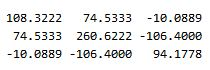X = MySample – repmat(mean(MySample),10,1);    % 中心化样本矩阵
C = (X’*X)./(size(X,1)-1)

（为方便对matlab不太明白的人，小小说明一下各个函数，同样，对matlab有一定基础的人直接跳过：

B = repmat(A,m,n ) %%将矩阵 A 复制 m×n 块，即把 A 作为 B 的元素，B 由 m×n 个 A 平铺而成。B 的维数是 [size(A,1)*m, (size(A,2)*n]

B = mean(A)的说明：

ans =
3.0000    4.5000    6.0000

ans =
2.0000
4.0000
6.0000

6.0000

size(A,n)%% 如果在size函数的输入参数中再添加一项n，并用1或2为n赋值，则 size将返回矩阵的行数或列数。其中r=size(A,1)该语句返回的是矩阵A的行数， c=size(A,2) 该语句返回的是矩阵A的列数）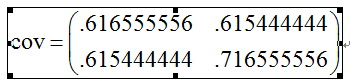3、计算协方差矩阵的特征向量和特征值

[eigenvectors,eigenvalues] = eig(cov)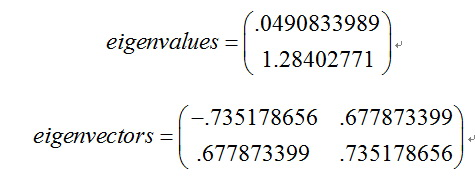4、选择成分组成模式矢量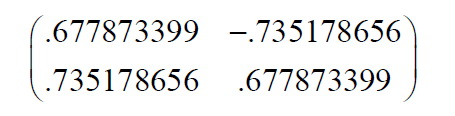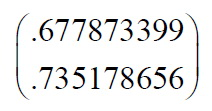5、得到降维后的数据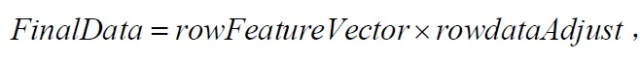（说明：如果要恢复原始数据，只需逆过程计算即可，即：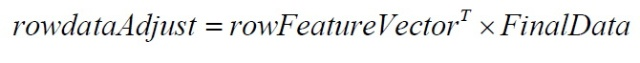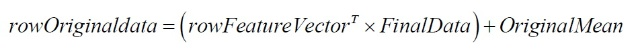15
36 收藏

### 作者的其它热门文章2019/09/04 10:56

#### 引用来自“偶提人间事”的评论

2018/08/21 10:47

2016/02/19 16:47

2014/04/22 22:28

4 评论
36 收藏
15